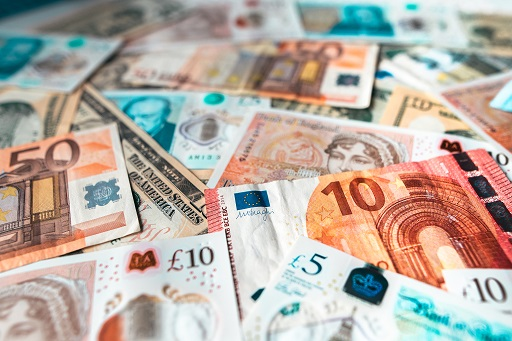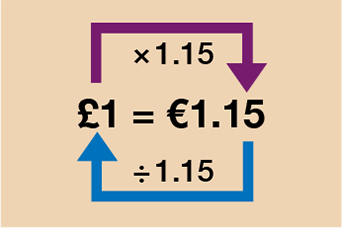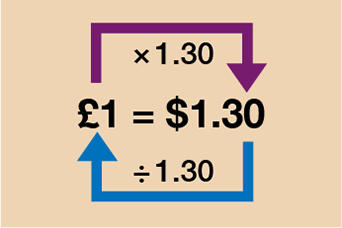Everyday maths 2 (Wales)

Start this free course now. Just create an account and sign in. Enrol and complete the course for a free statement of participation or digital badge if available.

# 2.1 Converting currencies

Often when you are abroad, or buying something from the internet from another country, the price will be given in a different currency. You’ll want to be able to work out how much the item costs in your own currency so that you can make a decision about whether to buy the item or not. When changing money for a holiday, you may also need to work out how much currency you will get for the amount of pounds sterling you are changing.Figure 7 Different currency notes

Let’s look at an example.

## Example: Pounds and euros

To convert between pounds and euros, the first thing you need to know is the exchange rate. Exchange rates change daily. If you are given a currency conversion question, though, you will be given the exchange rate to use. In this example we will use the exchange rate of:

• £1 = €1.15

So how do you convert?

Method

Look at the diagram below. In order to get from 1 to 1.15, you must multiply by 1.15. Following that, to get from 1.15 to 1, you must divide by 1.15.

In order to go from pounds to euros then, you multiply by 1.15 and to convert from euros to pounds, you divide by 1.15.Figure 8 A conversion rate for pounds and euros
• a.You decide you are going to change £300 into euros for your holiday. Using the exchange rate of £1 = €1.15, how many euros will you get?

Referring back to the diagram above, you want to convert pounds to euros in this example so you must multiply by 1.15.

However, you will not always have a diagram to refer to which means that you will need to be able to decide yourself whether you need to multiply by the conversion rate or divide by it. We will now look at the method to help you to do this.

Method

Write down your conversion rate with the single unit on the left-hand side:

• £1 = €1.15

Next, you write down the figure you are converting under the correct side. In this case it is the £300 cash you are changing, so this figure needs to go under the pounds (£) side:

• £1 = €1.15
• £300 = €?

This will help you to work out whether you need to go forwards (in which case you multiply by the conversion rate) or backwards (in which case you divide by the conversion rate).

Here, you are converting from pounds to euros. This means you are going forwards so you need to multiply by the conversion rate:

• £1 = €1.15
• £300 = €?
• forwards

Therefore, you must multiply by 1.15:

• £300 × 1.15 = €345

Let’s apply the method to another example.

• b.You are in Spain and want to buy a souvenir. The price is €35 (35 euros). You know that £1 = €1.15.

Method

Write the currency conversion rate down first with the single unit of measure (the 1) on the left hand-side.

• £1 = €1.15

Next, write down the figure you are converting under the correct side. In this case it is the cost of the souvenir (€35), so this figure needs to go on the euros (€) side:

• £1 = €1.15
• £? = €35

Here, you are converting from euros to pounds. This means you are going backwards so you need to divide by the conversion rate:

• £? = €35
• backwards ← therefore divide
• €35 ÷ 1.15 = £30.43 (rounded to two decimal places)

Before you try some for yourself, let’s look at one more example. This time involving pounds and dollars.

## Example: Pounds and dollars

• a.You want to buy an item from America. The cost is $120. You know that £1 =$1.30. How much does the item cost in pounds?

Method

Similarly to before, you can see from the diagram that in order to go from dollars to pounds you must divide by 1.30.Figure 9 A conversion rate for pounds and dollars

To work out $120 in pounds then, you do: •$120 ÷ 1.30 = £92.31 (rounded to two decimal places)

To figure this out without the aid of a diagram, write down your conversion rate:

• £1 = $1.30 Now write down the figure you want to convert (in this case the$120) under the correct side:

• £1 = $1.30 • £? =$120

As you are converting dollars to pounds, you are going backwards so need to divide by 1.30:

• $120 ÷ 1.30 = £92.31 (rounded to two decimal places) • b.You are sending a relative in America £20 for their birthday. You need to send the money in dollars. How much should you send? Method To convert from pounds to dollars, you need to write down you conversion rate: • £1 =$1.30

Now write down the figure you want to convert (in this case the £20) under the correct side:

• £1 = $1.30 • £20 =$?

As you are converting pounds to dollars, you are going forwards so need to multiply by 1.30:

• £20 × 1.30 = $26 You need to send$26.

## Summary of method

Write your conversion rate down first, making sure you put the single unit (the 1) on the left-hand side. This will make it easier to decide whether you need to multiply by the conversion rate or divide by it.

• If you are going forwards, you multiply by the conversion rate.

• If you are going backwards, you divide by the conversion rate.

## Activity 4: Currency conversions

Calculate the answers to the following questions without using a calculator. You may double-check your answers on a calculator if needed.

1. Sarah sees a handbag for sale on a cruise ship for €80. She sees the same handbag online for £65. She wants to pay the cheapest possible price for the bag. On that day the exchange rate is £1 = €1.25. Where should she buy the bag from?
2. Josh is going to South Africa and wants to change £250 into rand. He knows that £1 = 18.7 rand. How many rand will he get for his money?
3. Alice is returning from America and has $90 left over to change back into £s. Given the exchange rate of £1 =$1.27, how many £s will Alice receive? Give your answer rounded to two decimal places.

1. For this question you can either convert from £ to € or from € to £.

Converting from £ to €:

• £1 = €1.25
• £65 = €?

As you are converting pounds to euros, you are going forwards so need to multiply by 1.25:

• £65 × 1.25 = €81.25

Sarah should buy the bag on the ship.

Converting from € to £:

• £1 = €1.25
• £? = €80

As you are converting euros to pounds you are going backwards so need to divide by 1.25:

• €80 ÷ 1.25 = £64

Sarah should buy the bag on the ship.

2. You need to convert from £ to rand so you do:

• £1 = 18.7 rand
• £250 = ? rand

As you are converting pounds to rand you are going forwards so need to multiply by 18.7:

• £250 × 18.7 = 4675 rand

3. You need to convert from $to £ so you do: • £1 =$1.27

• £? = $90 As you are converting dollars to pounds, you are going backwards so need to divide by 1.27: •$90 ÷ 1.27 = £70.87 (rounded to two d.p.)

Hopefully you are now feeling confident with converting between currencies. The next part of this section deals with converting other units of measure between different systems – centimetres to inches, pounds to kilograms etc. These conversions are incredibly similar to converting currency and the conversion rate will always be given to you so it’s not something you have to remember.#### Find out more

*Eligibility rules apply for financial support.